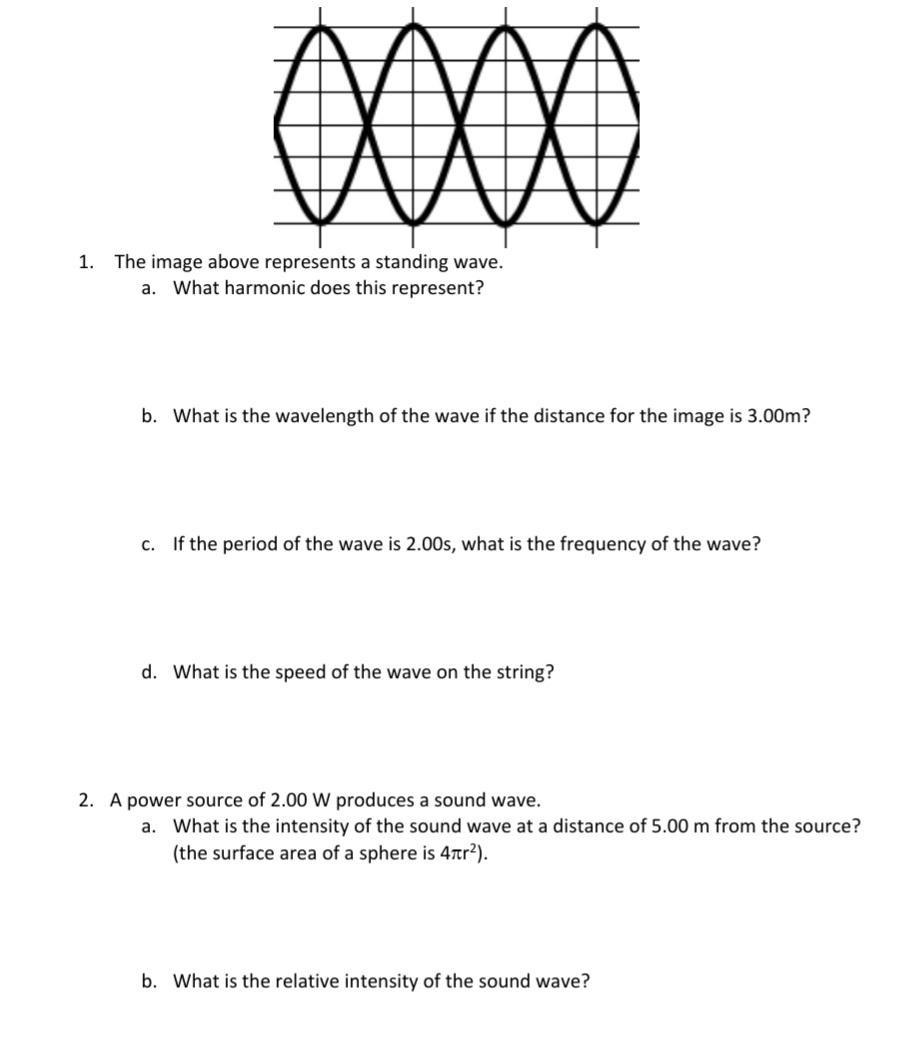Question:

# OOOO 1. The image above represents a standing wave. a. What harmonic does this represent? b. What is the wavelength of the waveOOOO 1. The image above represents a standing wave. a. What harmonic does this represent? b. What is the wavelength of the wave if the distance for the image is 3.00m? c. If the period of the wave is 2.00s, what is the frequency of the wave? d. What is the speed of the wave on the string? 2. A power source of 2.00 W produces a sound wave. a. What is the intensity of the sound wave at a distance of 5.00 m from the source? (the surface area of a sphere is 4tr2). b. What is the relative intensity of the sound wave?